# Seismic Design of Retaining WallReading time: 1 minute

Seismic design of retaining wall is considerably complicated problem in which assumptions have to be considered in order to make indeterminable issue solvable employing theory of statics and differential calculus. The computation of each static and dynamic pressure acting on retaining wall need more research and site selection is becoming codified. Site reports provide location peak ground acceleration only and utilization of this information is left for the designer.## When seismic design of retaining wall is required?

The code or standard that followed by designer play an important role in deciding whether the seismic design of retaining wall is required or not. The earthquake design necessity is argued by some. This is because, apart from waterfront wall where liquefaction is a possibility and walls which designed unsatisfactorily for static loads, evidence of retaining wall damage or deterioration approximately absent if the wall is designed perfectly. Furthermore, because retaining walls are not occupied consequently does not risk life safety except retaining walls that support buildings. However, International Building Code 2009 at section 1613 stated that every structure, and portion thereof, including nonstructural components that are permanently attached to structures and their supports and attachments, shall be designed and constructed to resist the effects of earthquake motions in accordance with ASCE 7. This obviously necessitate the design all structures for earthquake loads. Finally, it is advised that the designer check applicable Codes of the state in which specific seismic requirements are provided.

### Mononobe-Okabe Analysis of Earth Pressure

Mononobe-Okabe equation is the modification of coulomb equation and it considers seismic forces. The latter formula used to compute lateral earth pressure acts on retaining walls but it cannot be employed to calculate internal force which backfill soil impacts on the retaining walls during earthquake. The Mononobe-Okabe formula solve coulomb equation problem by taking both horizontal and vertical ground accelerations into consideration and provide seismic coefficients for passive (KPE) and active (KAE) pressure.And horizontal component is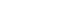Where: KAE: Active earth pressure coefficient, static +seismic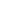: Slope of retaining wall to horizontal (90o used for vertical face)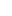: slope of backfill soil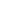: Angle of wall friction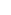:Angle of internal friction of soil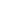: An angle whose tangent is the ground acceleration and computed by the following equation: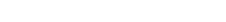Where: Kh: Horizontal ground acceleration When the face of the wall is vertical andis considered to be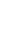, equation-1 becomes:Total force (active and earthquake) can be calculated by the following equation: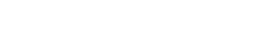Where: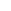: Soil density H: Height of retaining wall Furthermore, in case ground acceleration is equal to zero, KAE becomes the well-known coulomb KA equation. The passive earth pressure coefficient KPE is expressed as:It worth mentioning that passive pressure coefficient declines under seismic conditions. KAE Consist of two components includes static and seismic. The seismic (KAEKA) part is assumed to be an inverted triangular or trapezoidal diagram pressure with resultant force acting at 0.6H. moreover, (KA) is well known triangular distribution acting at (H/3). Furthermore, the location of combined resultant force is could be achieved employing the following equation: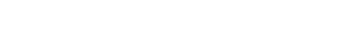It is suggested that the direction of force application per coulomb equation is tilt by an angle that is equal to the friction angle at the back face of the wall () which is considered to be, that is why the horizontal component is suggested to be: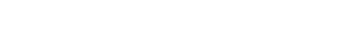Safety factor for sliding and overturning when earthquake is present is 1.1.

### Determining Lateral Seismic Earth Pressure (Kh)

Lateral seismic earth pressure which act against retaining wall is computed by equation Mononobe- Kobe formula using horizontal ground acceleration (Kh). This value is designed acceleration and is less severe compare with those accelerations that could occur at site. One third to one half of peak ground acceleration is employed if an arbitrary declined value of (Kh) is not utilized. The starting point is the determination of peak ground acceleration from Code such as International Building Code (IBC) 2009 and American Standard Society of Civil Engineer (ASCE) 7-10 both have similar charts. For 0.2 second select Maximum Considered Earthquake (MCE) ground motion from contours, spectral acceleration at 5 percent of critical damping, with 2 percent probability of exceedance in fifty years, note that the retaining walls are (short period) therefore 0.2 second selection. Read More: Loads and Forces Acting on Retaining Wall and Their Calculations